+

# Applying the Properties of Radicals

##### Rating:
(2)
• (0)
• (0)
• (1)
• (0)
• (1)
Author: Sophia Tutorial
##### Description:

Simplify a radical expression using the properties of radicals.

(more)
Tutorial
what's covered
1. Properties of Radicals
2. Cautions when Applying the Properties
3. Applying the Properties of Radicals

# 1. Properties of Radicals

There are several properties of radicals we can apply to simplify expressions involving radicals. The following properties are generally true whenever n is greater than 1, and a and b are both positive real numbers: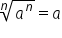and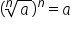Product Property: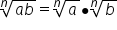Quotient Property: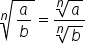Fractional Exponents:# 2. Cautions when Apply the Properties

Avoid these common errors when applying properties of radicals:

hint
The properties of radicals only apply to factors; they do not apply to terms. For example, we can use the product property of radicals to break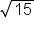into two radicals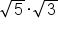because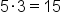. However, we cannot break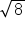into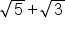.
hint
We can only bring an exponent outside of a radical if it applies to everything underneath the radical. For example, we can rewrite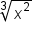as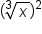because the exponent of 2 applied to everything underneath the radical. However,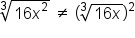This is because the exponent of 2 applies only to the x, not the 16. (We could rewrite the expression as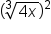because 16 =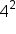)
hint
Taking the odd-root of a negative number leads to a real number solution, because a negative value raised to an odd exponent is negative. However, taking the even-root of a negative value leads to a non-real solution, because a negative value raised to an even exponent is never negative.

# 3. Applying the Properties of Radicals

When we recognize products, quotients, and powers with radicals, we can apply the properties of radicals to simplify the expression. This is shown in the examples below:

EXAMPLE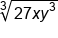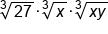Product property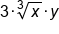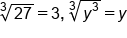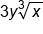Our Solution

EXAMPLE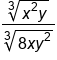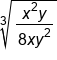Quotient property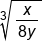One factor of x cancels; one factor of y cancels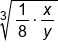Product Property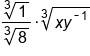Quotient property (and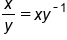Quotient property (and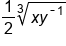Our Solution

summary
We can use the properties of radicals to simplify expressions and solve equations. There are some cautions when applying the properties. The properties of radicals apply only to factors, numbers, and variables combined by multiplication, not by addition or subtraction.

Formulas to Know
Product Property of RadicalsProperty of Fractional ExponentsQuotient Property of RadicalsRating Header